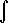# Maths - Clifford Calculus

## Prerequisites

If you are not familiar with quaternion algebra you may like to look at the following pages first:

## Clifford Differentiation with respect to Scalar

To differentiate a multivector with respect to a scalar, say 'x', we individually differentiate each element with respect to 'x'. So if:

f(x) = a + b e1 + c e2+ d e1^e2

then:

d f(x) / dx = d(a /dx) + d(b /dx) e1 + d(c /dx) e2+ d(d /dx) e1^e2

So this is quite simple, provided that we can differentiate the elements of a multivector, we can differentiate the whole multivector.

## Multivector Differentiation with respect to another Multivector

What are the rules of such differentiation? What applications does it have?

## Multivector Integration

As with differentiation we can integrate a whole multivector by individually integrating each element. So if:

f(x) = a + b e1 + c e2+ d e1^e2

then:f(x) dx =a dx +b dx e1 +c dx e2+d dx e1^e2